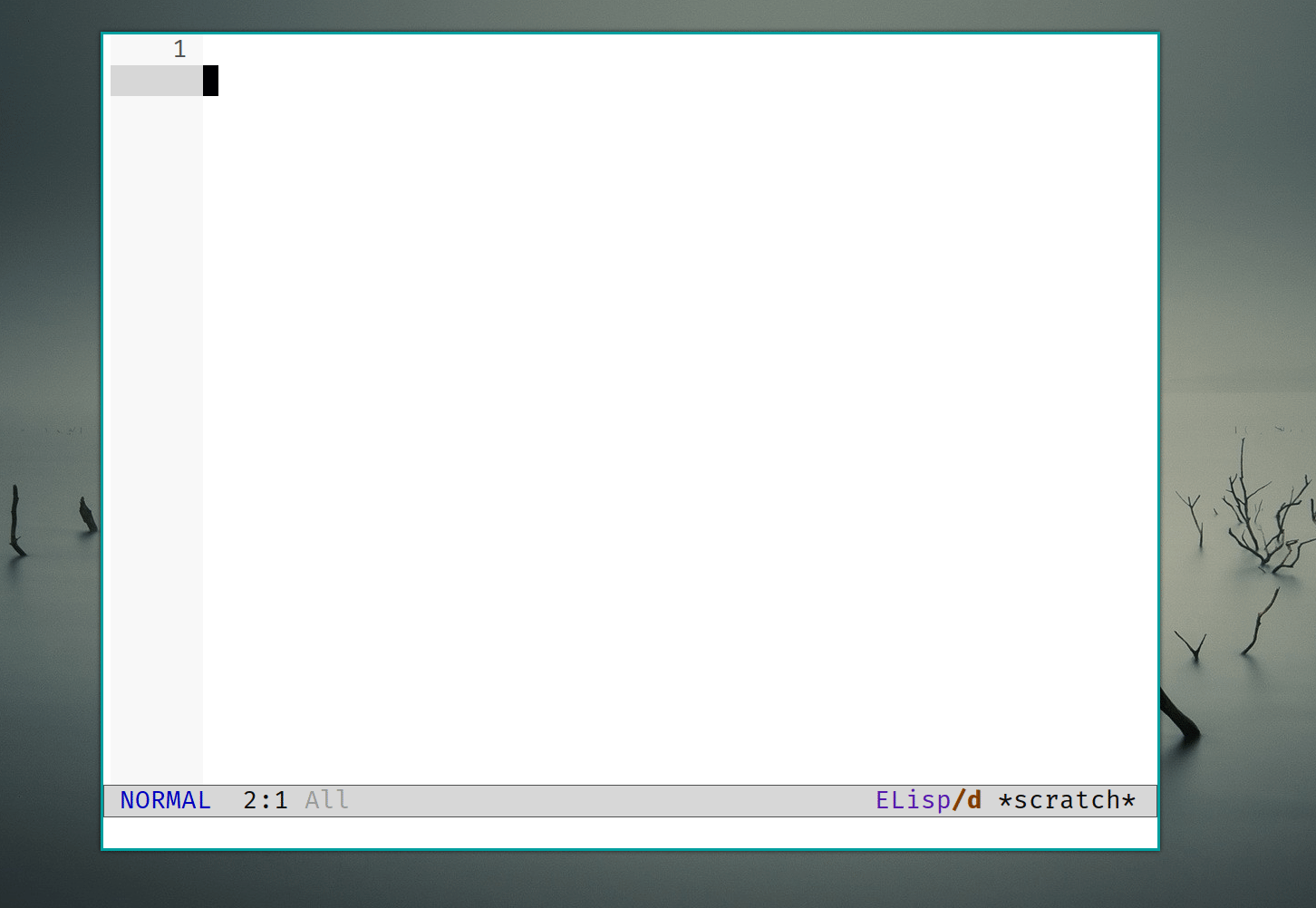# 写lisp时自动补全右括号的正确方法是？

``````(define (foo t)
(match t
[(abs fi x t1) (let ([new-ctx-x (pick-freshname ctx x)]))]))
^
``````

``````(define (foo t)
(match t
[(abs fi x t1) (let ([new-ctx-x (pick-freshname ctx x)]))]))
^
``````

1. 挨个数括号，并且多次按`C-f`
2. 并且根据括号对应的高亮提示来判断哪一个是正确的换行位置，从而继续写case。

``````(define (foo t)
(match t
[(abs fi x t1) (let ([new-ctx-x (pick-freshname ctx x)]))]
[]))
^
``````

``````(define (foo t)
(match t
[(abs fi x t1) (let ([new-ctx-x (pick-freshname ctx x
^
``````

1. 连续按`)`
2. 找到合适的位置然后换行
``````(define (foo t)
(match t
[(abs fi x t1) (let ([new-ctx-x (pick-freshname ctx x)]))]
[
^
``````

p.s. 贴一个很久之前发过的贴，这样关闭括号的方式反而让我感觉极其的轻松，很明确的知道自己在close哪。

``````(match expr
^
[pat1 body1]
[pat2 body2]
[pat2 body2])
``````

1 个赞

`paredit` 或者 `smartparens` 自动补全括号。1 个赞

``````(let ((l '(a b c d e)))
(-let (((x &rest y) l))
(progn
(let ())))
newline)
;; with super parent
(let [(l '(a b c d e]
[-let [((x &rest y) l]
(progn
(let (]
newline)
``````

1 个赞

You can either

``````(beginning-of-line)
(forward-sexp)
ENTER
``````

or

``````(evil-open-above)
input code
(end-of-line)
(transpose-sexps)``````

https://www.bilibili.com/video/BV1Pz4y1y7p9?p=4

1 个赞

super parent 这个概念不错。